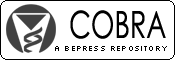## COBRA Preprint Series

#### Abstract

Censoring is a well known feature recurrent in the analysis of lifetime data, occurring in the model when exact lifetimes can be collected for only a representative portion of the surveyed individuals. If lifetimes are known only to exceed some given values, it is referred to as right censoring. In this paper we propose a systematization and a new derivation of the likelihood function for right censored sampling schemes; calculations are reported and assumptions are carefully stated. The sampling schemes considered (Type I, II and Random Censoring) give rise to the same ML function. Only the knowledge of elementary probability theory, namely the definitions of the order statistics and the conditional probability distribution function, are required in the proofs. Lastly we give an intuitive interpretation of Type I Censoring as a special case of Random Censoring, so that a global theory holds.

#### Disciplines

Statistical Methodology | Statistical Theory

COinS